# Orthogonal and Orthonormal Vectors in Linear Algebra

Orthogonal Vectors: Two vectors are orthogonal to each other when their dot product is 0.
How do we define the dot product?
Dot product(scalar product) of two n-dimensional vectors A and B, is given by this expression.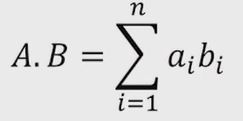Thus the vectors A and B are orthogonal to each other if and only if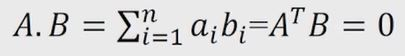Note: In a compact form the above expression can be wriiten as (A^T)B.

Example:
Consider the vectors v1 and v2 in 3D space.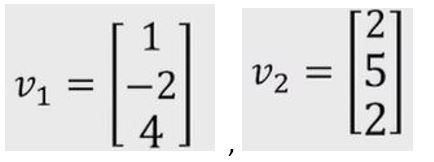Taking the dot product of the vectors.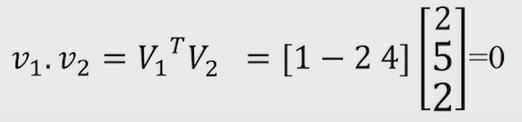Hence the vectors are orthogonal to each other.

Code: Python program to illustrate orthogonal vectors.

 `# A python program to illustrate orthogonal vector ` `  `  `# Import numpy module ` `import` `numpy ` `  `  `# Taking two vectors ` `v1 ``=` `[[``1``, ``-``2``, ``4``]] ` `v2 ``=` `[[``2``, ``5``, ``2``]] ` `  `  `# Transpose of v1 ` `transposeOfV1 ``=` `numpy.transpose(v1) ` `  `  `# Matrix multiplication of both vectors ` `result ``=` `numpy.dot(v2, transposeOfV1) ` `print``(``"Result  = "``, result) ` `  `  `# This code is contributed by Amiya Rout `

Output:

```Result = []
```

Unit Vector:
Let’s consider a vector A. The unit vector of the vector A may be defined as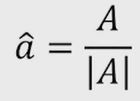Let’s understand this by taking an example. Consider a vector A in 2D space.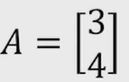The magnitude of A is given by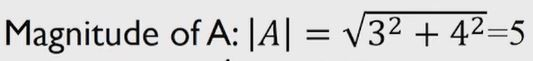So the unit vector of A can be calculated as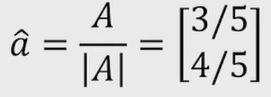Properties of unit vector:

• Unit vectors are used to define directions in a coordinate system.
• Any vectors can be written as a product of a unit vector and a scalar magnitude.

Orthonormal vectors:
These are the vectors with unit magnitude. Now, take the same 2 vectors which are orthogonal to each other and you know that when I take a dot product between these 2 vectors it is going to 0. So If we also impose the condition that we want each of these vectors to have unit magnitude then what we could possibly do is by taking this vector and then divide this vector by the magnitude of this vector as we see in unit vector. Now we can write v1 and v2 as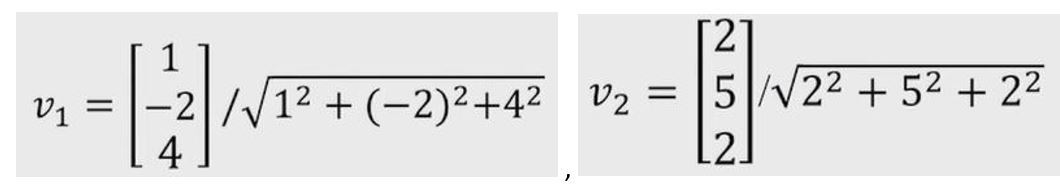So what we do is we have taken the vectors from the previous example and converted them into unit vectors by dividing them with their magnitudes. So, these vectors will still be orthogonal to each other and now individually they also have unit magnitude. Such vectors are known as orthonormal vectors.

Note: All orthonormal vectors are orthogonal by the definition itself.

Don’t stop now and take your learning to the next level. Learn all the important concepts of Data Structures and Algorithms with the help of the most trusted course: DSA Self Paced. Become industry ready at a student-friendly price.

My Personal Notes arrow_drop_upCheck out this Author's contributed articles.

If you like GeeksforGeeks and would like to contribute, you can also write an article using contribute.geeksforgeeks.org or mail your article to contribute@geeksforgeeks.org. See your article appearing on the GeeksforGeeks main page and help other Geeks.

Please Improve this article if you find anything incorrect by clicking on the "Improve Article" button below.

Article Tags :

Be the First to upvote.

Please write to us at contribute@geeksforgeeks.org to report any issue with the above content.